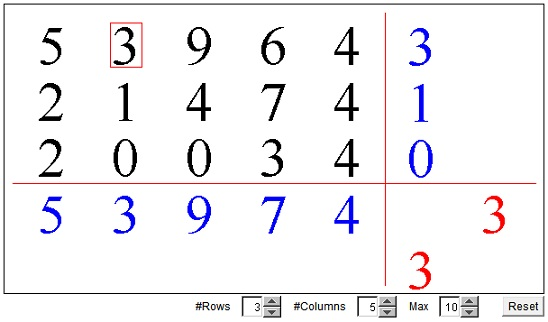# Minimax Principle

The purpose of the applet below is to illustrate a mathematical fact that plays an important role in the Game Theory, Economics, and general optimization problems. I postpone the statement until later. See if, with the help of the applet, you can arrive at the right formulation yourself.

Given a rectangular array of numbers. In every column, select the maximum value and write it under the column. Similarly, in every row, select the minimum value and write it to the right of the column. In the applet, these computed values appear in blue. In this fashion, our original array of numbers is augmented with one (blue) row and one (blue) column. In the blue row select the minimum element and write it (in red) to the right of the row. Similarly, in the blue column select the maximum number and right it under that column. Compare the two (red) numbers. Is there any regularity? Most of the time the numbers will be different. Sometimes they will coincide. On such occasions, the applet will provide an additional clue. Try to grasp its significance.

To get a different configuration, press the Reset button. With larger matrix dimensions, it may become tiresome to keep pressing the Reset, just in order to get a matrix with a saddle point. However, each of the numbers in the matrix can be changed individualy. Click or drag the mouse off the vertical center line of each entry. The number will go down or up, depending on whether the cursor is to the left or right of the central line.

### If you are reading this, your browser is not set to run Java applets. Try IE11 or Safari and declare the site https://www.cut-the-knot.org as trusted in the Java setup.What if applet does not run?

StatementCopyright © 1996-2018 Alexander Bogomolny

Let's first of all formalize the procedure. We start with an array Ai,k of numbers. By convention, the first index designate a row, the second a column. For example, element A3,5 is located in the row number 3 and the column # 5. Keep the first index fixed - this is to say, select a row (the row corresponding to the fixed first index.) Denote the minimum element in this row as Bi = Min(Ai,k). (The minimum is taken over all k for a fixed i.) The result generally depends on the selected row. Denote the maximum number among Bi's as MaxMin = Max(Bi). (The maximum is taken over all i's.)

In a similar manner, let Ck = Max(Ai,k), where, as before, maximum is taken over all i's (for a fixed k, i.e., for a fixed column.) Compute then MinMax = Min(Ck).

### Statement

1. MinMax ≥ MaxMin
2. The equality is achieved iff there exists an element Ai0,k0 that is simultaneously maximal in its column and minimal in its row.

### Proof

Writing subscripts in HTML (this is the language of the Web) is somewhat awkward. Let's just agree that Max is taken over index i, whereas Min is always taken over index k.

Assume MinMax = Ai0 ,k0 for some indices i0 and k0. MinMax = Min(Ck) = Ck0. In other words, MinMax is the maximum element in column k0. By definition, Ck0 = Max(Ai, k0). Every element Ai,k0 in column k0 may or may not be the smallest number in its row. But, in any event, the smallest number in a row can't exceed any element in that row:

Ai,k0 ≥ Min(Ai, k) = Bi

Combining that with

Ai0, k0 = Max(Ai, k0) ≥ Ai, k0

(which is just a different way of describing our selection of indices) we get

Ai0,k0 ≥ Min(Ai,k) = Bi

The left hand side in the latter does not depend on the index i, and the inequality holds for any value of i (any row in the table.) Therefore, maximizing the right hand side will preserve the inequality:

 (1) MinMax = Ai0,k0 ≥ Max(Bi) = MaxMin

which proves the first part of the statement.

To prove the second part, first assume that MinMax = MaxMin. Introduce

MinMax = Min(Ck) = Ck0 and
MaxMin = Max(Bi) = Bi0,

and consider Ai0,k0. By definition,

MaxMin = Bi0 ≤ Ai0,k0 ≤ Ck0 = MinMax.

By the assumption MinMax = MaxMin,

MinMax = Ai0,k0 = MaxMin.

Conversely, assume there exists an element Ai0,k0 such that

Ck0 = Max(Ai, k0) = Ai0, k0 = Min(Ai0, k) = Bi0

Then, by definition,

MaxMin = Max(Bi) ≥ Bi0 = Ck0 ≥ Min(Ck) = MinMax

However, as we have shown in the first part of the proof, MinMax ≥ MaxMin always, which gives MinMax = MaxMin.The point (i0, k0) such that MinMax = Ai0,k0 = MaxMin is called a saddle point for a given table A. Saddle points may exist for functions of continuous arguments as well. Graphs of such functions in a vicinity of a saddle point in fact resemble the shape of a saddle.

The saddle points have interesting properties that you may observe with the applet and also try proving:

1. If there are more than one saddle point, then all corresponding matrix entries coincide.
2. If there are two saddle points at (i1, j1) and (i2, j2), such that i1 differs from i2 and j1 differs from j2, then (i1, j2) and (i2, j1) are also saddle points.

Peter Bajorski rewrote the above replacing matrices with a more general functional setting. His writeup is available as a pdf file.### A Sample of Optimization Problems II

• Mathematicians Like to Optimize
• Building a Bridge
• Building Bridges
• Optimization Problem in Acute Angle
• Sangaku with Quadratic Optimization
• Geometric Optimization from the Asian Pacific Mathematical Olympiad
• Cassini's Ovals and Geometric Optimization
• Heron's Problem
• Optimization in Parallelepiped
• Matrices and Determinants as Optimization Tools: an Example
• An Inequality between AM, QM and GM
•Copyright © 1996-2018 Alexander Bogomolny

 65250409